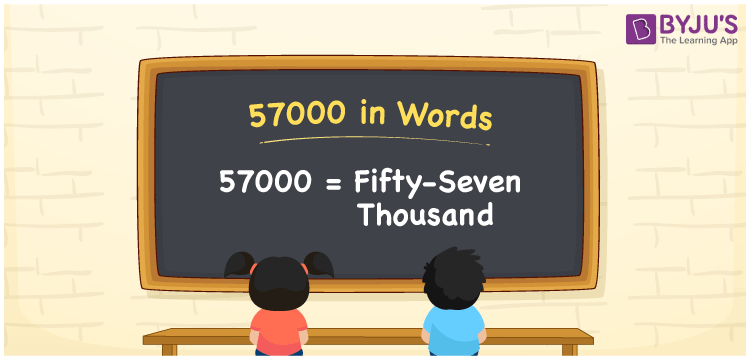# 57000 in Words

57000 in words is written as Fifty-seven thousand. The name of 57,000 in English is Fifty-seven thousand. The number 57000 represents a value equivalent to the original number. For example, a person has 57,000 rupees in his bank account, so it is expressed as Fifty-seven thousand rupees in words. Even while writing a cheque of rupees 57000, it is written as Fifty-seven thousand rupees only or Fifty-seven thousand only. Hence, it is necessary for students to learn how to read and write the numbers in words. To express 57000 in words, we have to check the place values of individual digits. Let us learn how to write the number 57000 in words, in this article.

 57000 in words Fifty-seven thousand Fifty-seven thousand in Numbers 57000

## 57000 in English Words## How to Write 57000 in Words?

Below, you will learn how the number 57000 can be written in words. There are five digits in the number 57000, so let us prepare a place value chart which shows the place value for all the four digits.

 Ten thousands Thousands Hundreds Tens Ones 5 7 0 0 0

We can write it in expanded form as:

5 × Ten thousand + 7 × Thousand + 0 × Hundred + 0 × Ten + 0 × One

= 5 × 10000 + 7 × 1000  + 0 × 100 + 0 × 10 + 0 × 1

= 50000 + 7000

= 57000

= Fifty-seven thousand

Therefore, 57000 in words is written as Fifty-seven thousand.

57000 is a natural number that precedes 57001 and succeeds 56999.

57000 in words – Fifty-seven thousand

Is 57000 an odd number? – No

Is 57000 an even number? – Yes

Is 57000 a perfect square number? – No

Is 57000 a perfect cube number? – No

Is 57000 a prime number? – No

Is 57000 a composite number? – Yes

## Frequently Asked Questions on 57000 in Words

Q1

### How to write 57000 in words?

57000 in English is written as “Fifty-seven thousand”.
Q2

### Is 57000 an odd number or even number?

57000 is an even number because it is wholly divisible by 2.
57000/2 = 28500
Q3

### Write Fifty-seven thousand in numbers.

Fifty-seven thousand in numbers is 57000.